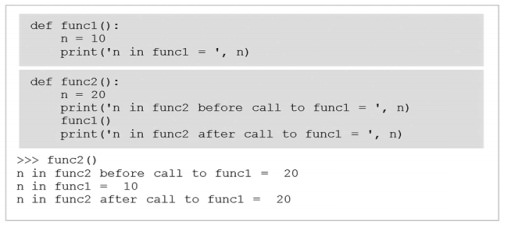Home | | Problem Solving and Python Programming | Parameters and arguments - Python

# Parameters and arguments - Python

Some of the built-in functions we have seen require arguments.

Parameters and arguments

Some of the built-in functions we have seen require arguments. For example, when you call math.sin you pass a number as an argument. Some functions take more than one argument: math.pow takes two, the base and the exponent.

Inside the function, the arguments are assigned to variables called parameters. Here is an example of a user-defined function that takes an argument:

Eg: def print_twice(bruce):

print bruce

print bruce

This function assigns the argument to a parameter named bruce. When the function is called, it prints the value of the parameter (whatever it is) twice.

This function works with any value that can be printed.

>>> print_twice('Spam')

Spam

Spam

>>> print_twice(17)

17

17

>>> print_twice(math.pi)

3.14159265359

3.14159265359

## Parameter Passing

Parameter passing is the process of passing arguments to a function. There are two types of arguments: Actual arguments and formal arguments. Actual arguments are the values passed to a function’s formal parameters to be operated on.

Eg:

def f(x): #name x used as formal parameter

y = 1

x = x + y

print 'x =', x

return x

x = 3

y = 2

z = f(x) #value of x used as actual parameter

print 'z =', z

print 'x =', x

print 'y =', y

When run, this code prints,

x = 4

z = 4

x = 3

y = 2

A default argument is an argument that can be optionally provided in a given function call. When not provided, the corresponding parameter provides a default value.

Eg:

def greet(name, msg = "Good morning!"):

"""

This function greets to

the person with the

provided message.

If message is not provided,

it defaults to "Good

morning!"

"""

print("Hello",name + ', ' + msg)

greet("Kate")

greet("Bruce","How do you do?")

Output:

Hello Kate, Good morning!

Hello Bruce, How do you do?

## Scope and Lifetime of variables

Scope of a variable is the portion of a program where the variable is recognized. Parameters and variables defined inside a function is not visible from outside. Hence, they have a local scope.

Lifetime of a variable is the period throughout which the variable exits in the memory.

The lifetime of variables inside a function is as long as the function executes.

They are destroyed once we return from the function. Hence, a function does not remember the value of a variable from its previous calls.

Eg:

def my_func():

x = 10

print("Value inside function:",x)

x = 20

my_func()

print("Value outside function:",x)

Output:

Value inside function: 10

Value outside function: 20

## Local Scope and Local Variables

A local variable is a variable that is only accessible from within a given function. Such variables are said to have local scope .## Global Variables and Global Scope

A global variable is a variable that is defined outside of any function definition. Such variables are said to have global scope.Variable max is defi ned outside func1 and func2 and therefore “global” to each.

Study Material, Lecturing Notes, Assignment, Reference, Wiki description explanation, brief detail
Problem Solving and Python Programming : Data, Expressions, Statements : Parameters and arguments - Python |